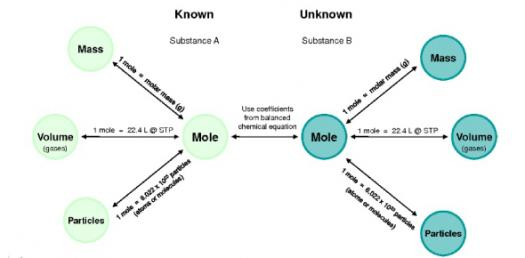# An Easy Quiz On Stoichiometry

10 Questions | Total Attempts: 388SettingsStoichiometry is the calculation that deals with the amount of reactants required and the amount of products formed during a chemical reaction. It is based on the Law of Conservation of Mass according to which the total mass before and after a chemical reaction remains undisturbed. The following quiz is an easy stoichiometry test. Balance all equations before solving. Good Luck!

• 1.
Na + Cl2 --> NaCl (unbalanced) How many moles of chlorine gas (Cl2) would react with 5.0 moles of sodium (Na)        according to the following chemical equation?
• A.

1.0

• B.

1.5

• C.

2.0

• D.

2.5

• E.

3.0

• 2.
Na + Cl2 --> NaCl (unbalanced) Using the equation (after it is balanced) above, determine the amount of product that          can be produced  from 24.7 g Na.  (in grams)
• A.

55

• B.

62

• C.

71

• D.

82

• E.

84

• 3.
In the reaction C8H18 + O2 --> CO2 + H2O (unbalanced) If 27.3g of C8H18 are combusted, what mass of water will be produced?( in grams)
• A.

13.5

• B.

23.4

• C.

53.2

• D.

47.1

• E.

37.8

• 4.
CH4   +   O2  -->  CO2   +   2 H2O How many moles of O2 are required to burn 0.5 moles of CH4?
• A.

1

• B.

2

• C.

3

• D.

4

• E.

5

• 5.
NaOH   +   HCl   --> NaCl   +   H2O How many grams of NaOH will react with 36.5 g of HCl? ( in grams)
• A.

40

• B.

60

• C.

80

• D.

100

• E.

120

• 6.
How many moles of water is formed from the combustion of 5 moles of hydrogen gas in oxygen gas?
• A.

2.5

• B.

4

• C.

5

• D.

7

• E.

None of the above

• 7.
Methane gas (CH4) gets burned in oxygen (O2) to form carbon dioxide (CO2) and water vapor (H2O). The balanced reaction is
• A.

CH4 --> CO2 + 2H2O

• B.

2CH4 + O2 --> 2CO2 + 2H2O

• C.

CH4 + 2O2 --> CO2 + 2H2O

• D.

3CH4 + 2O2 --> CO2 + 2H2O

• E.

4CH4 + 2O2 --> 4CO2 + 2H2O

• 8.
Methane gas (CH4) gets burned in oxygen (O2) to form carbon dioxide (CO2) and water vapor (H2O). If I burn 10 grams of methane, how many grams of CO2 will be produced?
• A.

42.7 grams of CO2

• B.

833 grams of CO2

• C.

20 grams of CO2

• D.

27.4 grams of CO2

• E.

30 grams of CO2

• 9.
In the decomposition of water to hydrogen gas and oxygen gas, how many moles of water is needed to produce 10.0 moles of oxygen gas?
• A.

5.00

• B.

10.0

• C.

15.0

• D.

20.0

• E.

25.0

• 10.
In the decomposition of water to hydrogen gas and oxygen gas, how many moles of water is needed to produce 32 grams of oxygen gas?
• A.

0.5

• B.

1.0

• C.

1.5

• D.

2.0

• E.

2.5

Related TopicsBack to top